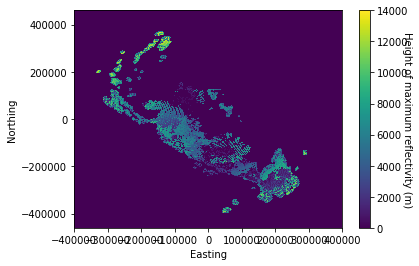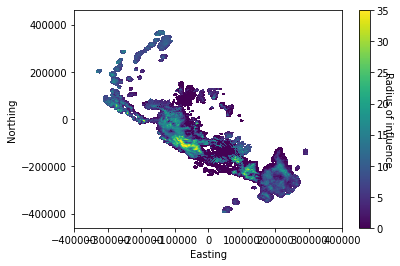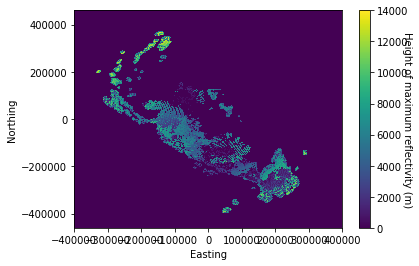#### Notebooks

##### NZ radar raw data connectorCategory:
Author:

##### Content:
###### Python libraries

We install pyart which is a python library specilised in dealing with raw radar data. We also load matplotlib which will be used to generate plots.

In :

``````import sys
!{sys.executable} -m pip install arm-pyart matplotlib
``````
``````Collecting arm-pyart
Collecting matplotlib
Using cached https://files.pythonhosted.org/packages/93/4b/52da6b1523d5139d04e02d9e26ceda6146b48f2a4e5d2abfdf1c7bac8c40/matplotlib-3.2.1-cp36-cp36m-manylinux1_x86_64.whl
Collecting cycler>=0.10 (from matplotlib)
Using cached https://files.pythonhosted.org/packages/f7/d2/e07d3ebb2bd7af696440ce7e754c59dd546ffe1bbe732c8ab68b9c834e61/cycler-0.10.0-py2.py3-none-any.whl
Collecting pyparsing!=2.0.4,!=2.1.2,!=2.1.6,>=2.0.1 (from matplotlib)
Collecting kiwisolver>=1.0.1 (from matplotlib)
Collecting numpy>=1.11 (from matplotlib)
Collecting python-dateutil>=2.1 (from matplotlib)
Using cached https://files.pythonhosted.org/packages/d4/70/d60450c3dd48ef87586924207ae8907090de0b306af2bce5d134d78615cb/python_dateutil-2.8.1-py2.py3-none-any.whl
Collecting six (from cycler>=0.10->matplotlib)
Using cached https://files.pythonhosted.org/packages/65/eb/1f97cb97bfc2390a276969c6fae16075da282f5058082d4cb10c6c5c1dba/six-1.14.0-py2.py3-none-any.whl
Collecting setuptools (from kiwisolver>=1.0.1->matplotlib)
Using cached https://files.pythonhosted.org/packages/a0/df/635cdb901ee4a8a42ec68e480c49f85f4c59e8816effbf57d9e6ee8b3588/setuptools-46.1.3-py3-none-any.whl
Installing collected packages: arm-pyart, six, cycler, pyparsing, setuptools, kiwisolver, numpy, python-dateutil, matplotlib
Successfully installed arm-pyart-1.11.1 cycler-0.10.0 kiwisolver-1.1.0 matplotlib-3.2.1 numpy-1.18.2 pyparsing-2.4.6 python-dateutil-2.8.1 setuptools-46.1.3 six-1.14.0``````

In :

``````import pyart
from matplotlib import pyplot as plt
import numpy as np``````
``````## You are using the Python ARM Radar Toolkit (Py-ART), an open source
## library for working with weather radar data. Py-ART is partly
## supported by the U.S. Department of Energy as part of the Atmospheric
## Radiation Measurement (ARM) Climate Research Facility, an Office of
## Science user facility.
##
## If you use this software to prepare a publication, please cite:
##
##     JJ Helmus and SM Collis, JORS 2016, doi: 10.5334/jors.119``````
``````/usr/local/lib/python3.6/dist-packages/_pytest/mark/structures.py:370: DeprecationWarning: The usage of `cmp` is deprecated and will be removed on or after 2021-06-01.  Please use `eq` and `order` instead.
@attr.s(cmp=False, hash=False)
``````

The New Zealand MetService's radar data are generated by a network of 8 doppler radars spread around New Zealand. Each radar does a scan of it's surroundings every 7 to 8 minutes. The radar operate independantly and produce one file for every scan.

Let's read one of the example files and look at the structure of the data. First we use pyart to load the data in the shape of a radar object.

In :

``````file = './sample_data/BOP170101000556.RAWSURV'

The radar files contain polar data from two sweeps (two revolution of the radar beam each for a different vertical angle). Each sweep contains data over a number of rays (angle) and gates (segments in the radial direction). Informations about the content of the file can be found using the info function of the radar object.

In :

``radar.info()``
``````altitude:
data: <ndarray of type: float64 and shape: (1,)>
long_name: Altitude
standard_name: Altitude
units: meters
positive: up
altitude_agl: None
antenna_transition: None
azimuth:
data: <ndarray of type: float32 and shape: (840,)>
units: degrees
standard_name: beam_azimuth_angle
long_name: azimuth_angle_from_true_north
comment: Azimuth of antenna relative to true north
elevation:
data: <ndarray of type: float32 and shape: (840,)>
units: degrees
standard_name: beam_elevation_angle
long_name: elevation_angle_from_horizontal_plane
comment: Elevation of antenna relative to the horizontal plane
fields:
total_power:
data: <ndarray of type: float32 and shape: (840, 1000)>
units: dBZ
standard_name: equivalent_reflectivity_factor
long_name: Total power
coordinates: elevation azimuth range
_FillValue: -9999.0
reflectivity:
data: <ndarray of type: float32 and shape: (840, 1000)>
units: dBZ
standard_name: equivalent_reflectivity_factor
long_name: Reflectivity
coordinates: elevation azimuth range
_FillValue: -9999.0
fixed_angle:
data: <ndarray of type: float32 and shape: (2,)>
long_name: Target angle for sweep
units: degrees
standard_name: target_fixed_angle
instrument_parameters:
unambiguous_range:
data: <ndarray of type: float32 and shape: (840,)>
units: meters
meta_group: instrument_parameters
long_name: Unambiguous range
prt_mode:
data: <ndarray of type: |S5 and shape: (2,)>
comments: Pulsing mode Options are: "fixed", "staggered", "dual". Assumed "fixed" if missing.
meta_group: instrument_parameters
long_name: Pulsing mode
units: unitless
prt:
data: <ndarray of type: float32 and shape: (840,)>
units: seconds
comments: Pulse repetition time. For staggered prt, also see prt_ratio.
meta_group: instrument_parameters
long_name: Pulse repetition time
prt_ratio:
data: <ndarray of type: float32 and shape: (840,)>
units: unitless
meta_group: instrument_parameters
long_name: Pulse repetition frequency ratio
nyquist_velocity:
data: <ndarray of type: float32 and shape: (840,)>
units: meters_per_second
meta_group: instrument_parameters
long_name: Nyquist velocity
data: <ndarray of type: float32 and shape: (1,)>
units: degrees
long_name: Antenna beam width H polarization
data: <ndarray of type: float32 and shape: (1,)>
units: degrees
long_name: Antenna beam width V polarization
pulse_width:
data: <ndarray of type: float32 and shape: (840,)>
units: seconds
meta_group: instrument_parameters
long_name: Pulse width
latitude:
data: <ndarray of type: float64 and shape: (1,)>
long_name: Latitude
standard_name: Latitude
units: degrees_north
longitude:
data: <ndarray of type: float64 and shape: (1,)>
long_name: Longitude
standard_name: Longitude
units: degrees_east
nsweeps: 2
ngates: 1000
nrays: 840
range:
data: <ndarray of type: float32 and shape: (1000,)>
units: meters
standard_name: projection_range_coordinate
long_name: range_to_measurement_volume
spacing_is_constant: true
comment: Coordinate variable for range. Range to center of each bin.
meters_to_center_of_first_gate: [0.]
meters_between_gates: [300.]
scan_rate: None
scan_type: ppi
sweep_end_ray_index:
data: <ndarray of type: int32 and shape: (2,)>
long_name: Index of last ray in sweep, 0-based
units: count
sweep_mode:
data: <ndarray of type: |S20 and shape: (2,)>
units: unitless
standard_name: sweep_mode
long_name: Sweep mode
comment: Options are: "sector", "coplane", "rhi", "vertical_pointing", "idle", "azimuth_surveillance", "elevation_surveillance", "sunscan", "pointing", "manual_ppi", "manual_rhi"
sweep_number:
data: <ndarray of type: int32 and shape: (2,)>
units: count
standard_name: sweep_number
long_name: Sweep number
sweep_start_ray_index:
data: <ndarray of type: int32 and shape: (2,)>
long_name: Index of first ray in sweep, 0-based
units: count
target_scan_rate: None
time:
data: <ndarray of type: float64 and shape: (840,)>
units: seconds since 2017-01-01T00:05:56Z
standard_name: time
long_name: time_in_seconds_since_volume_start
calendar: gregorian
comment: Coordinate variable for time. Time at the center of each ray, in fractional seconds since the global variable time_coverage_start
version: 1.3
title:
institution:
references:
source:
history:
comment:
original_container: sigmet
time_ordered: none
rays_missing: 0``````
###### Plotting the raw data

The raw data from the two sweeps are stored in a single two dimensional array. They can be plotted in the form of two polar plots.

In :

``````%matplotlib inline

## Create nsweeps polar plots
fig, axs = plt.subplots(1, radar.nsweeps, subplot_kw=dict(polar=True))

## For each plot find start and end indices
# Convert azimuth to geometric angle

# Create grid from indices

# Plot data on grid

cbar_ax = fig.add_axes([0.85, 0.15, 0.05, 0.7])
cbar = fig.colorbar(im, cax=cbar_ax)
cbar.set_label("Reflectivity (dBZ)", rotation=270)``````Those two plots allow to get a good idea of the horizontal spreading of the reflectivity field. However the data recorded by the radar is three-dimensional and slightly spread over time. Indeed, as shows below it takes about 1 minute for the two sweeps to be recorded.

In :

``````%matplotlib inline

plt.ylabel("Time since start of scan (s)")
plt.xlabel("ID for ray")``````

Out:

``Text(0.5, 0, 'ID for ray')``In :

``````## Path to the 2 files to collate

## Set filters for the radar object
filters = []
threshold=-100, size=20)
gatefilter.exclude_transition()
gatefilter.exclude_above('reflectivity', 80)
filters.append(gatefilter)

## Do regridding
grid_shape= (41, 2250, 2000),
grid_limits= ([0.0, 20000.0], [-460000.0, 460000.0], [-400000.0, 400000.0]),
fields=['reflectivity'],
max_refl= 80.,
copy_field_data= True,
grid_origin= (-37.0, 175.5),
roi_func= 'dist_beam',
h_factor= 1.0,
nb= 1.0,
bsp= 1.0
)
``````
``````/home/sebastien/.local/lib/python3.6/site-packages/pyart/map/gates_to_grid.py:162: DeprecationWarning: Barnes weighting function is deprecated. Please use Barnes 2 to be consistent with Pauley and Wu 1990.
" Pauley and Wu 1990.", DeprecationWarning)``````

Now we can plot five cross-sections of the gridded data at 5 fixes vertical levels (every 10 vertical levels of the grid). And get an idea of the 3D structure of the data.

In :

``````%matplotlib inline
fig, axs = plt.subplots(1, 5)

for ax, data in zip(axs.flat, grid.fields['reflectivity']['data'][::10,:,:]):
ax.pcolor(data, vmin=0, vmax=35)``````We can also plot cross-sections along the north-south and east-west directiontp get an idea of the vertical structure of the data

In :

``````%matplotlib inline

fig, axs = plt.subplots(1, 2)

ax = axs.flat
ax.pcolor(grid.x['data']/1000., grid.z['data'][:30]/1000.,
grid.fields['reflectivity']['data'][:30,1125,:])
ax.set(xlabel="Distance from radar", ylabel="Height agl (km)")

ax = axs.flat
plot = ax.pcolor(grid.y['data']/1000., grid.z['data'][:30]/1000.,
grid.fields['reflectivity']['data'][:30,:,1000])

Out:

``[Text(0.5, 0, 'Distance from radar')]``3D data can be quite heavy to work with and something one only consider the maximum reflectivity over the atmospheric column.

In :

``````%matplotlib inline

plt.pcolor(grid.x['data'], grid.y['data'],
np.amax(grid.fields['reflectivity']['data'][:,:,:], axis=0), vmin=0, vmax=35)
plt.xlabel('Easting')
plt.ylabel('Northing')
cbar = plt.colorbar()One can always keep some information on the vertical structure by storing the height at which the maximum in reflectivity was found

In :

``max_reflectivity_height = grid.z['data'][np.argmax(grid.fields['reflectivity']['data'][:,:,:], axis=0)]``

In :

``````%matplotlib inline

plt.pcolor(grid.x['data'], grid.y['data'], max_reflectivity_height)
plt.xlabel('Easting')
plt.ylabel('Northing')
cbar = plt.colorbar()
cbar.set_label("Height of maximum reflectivity (m)", rotation=270)``````An additional field that is produced during the regridding process is the radius of influence. This quantify the distance between the data point in the grid and the instrument that recorded it. This is an important quantity as the resolution and hence quality of the data drops with the distance from the instrument. On the plot below one can clearly see that two sources were used to created the grid, their location and how the radius of influence increases with the distance from the radars.

In :

``````%matplotlib inline

plt.pcolor(grid.x['data'], grid.y['data'], grid.fields['ROI']['data'][0,:,:])
plt.xlabel('Easting')
plt.ylabel('Northing')
cbar = plt.colorbar()